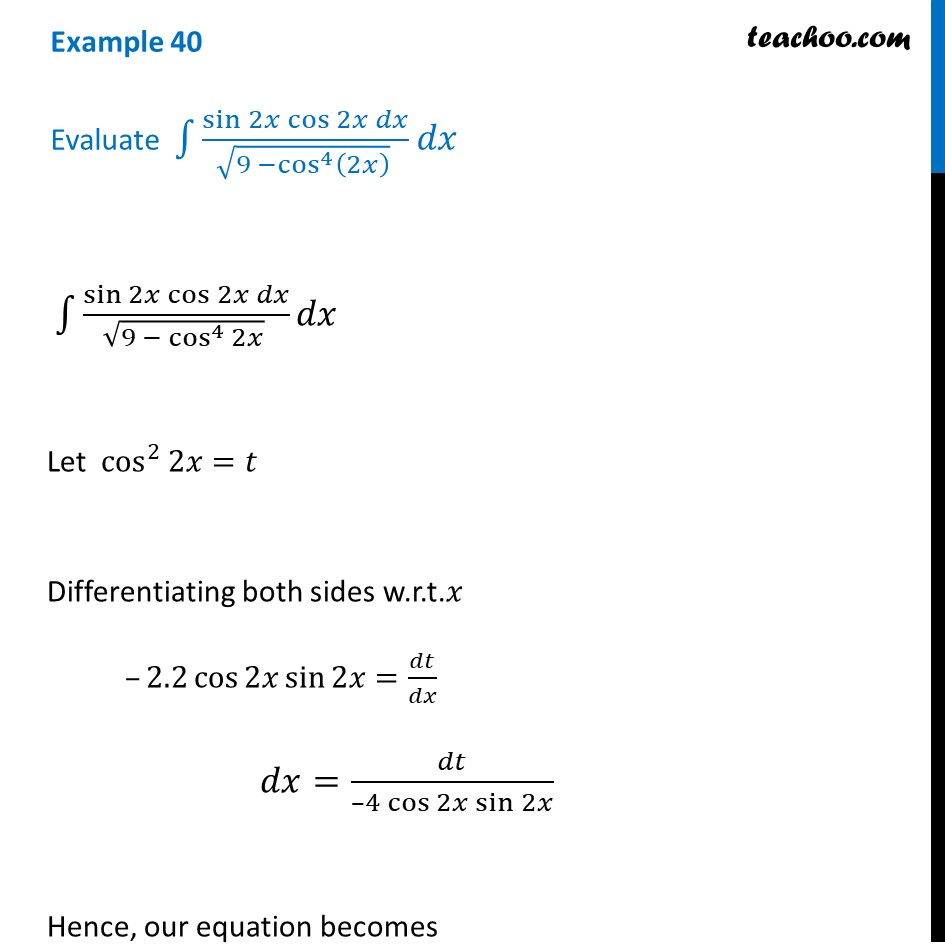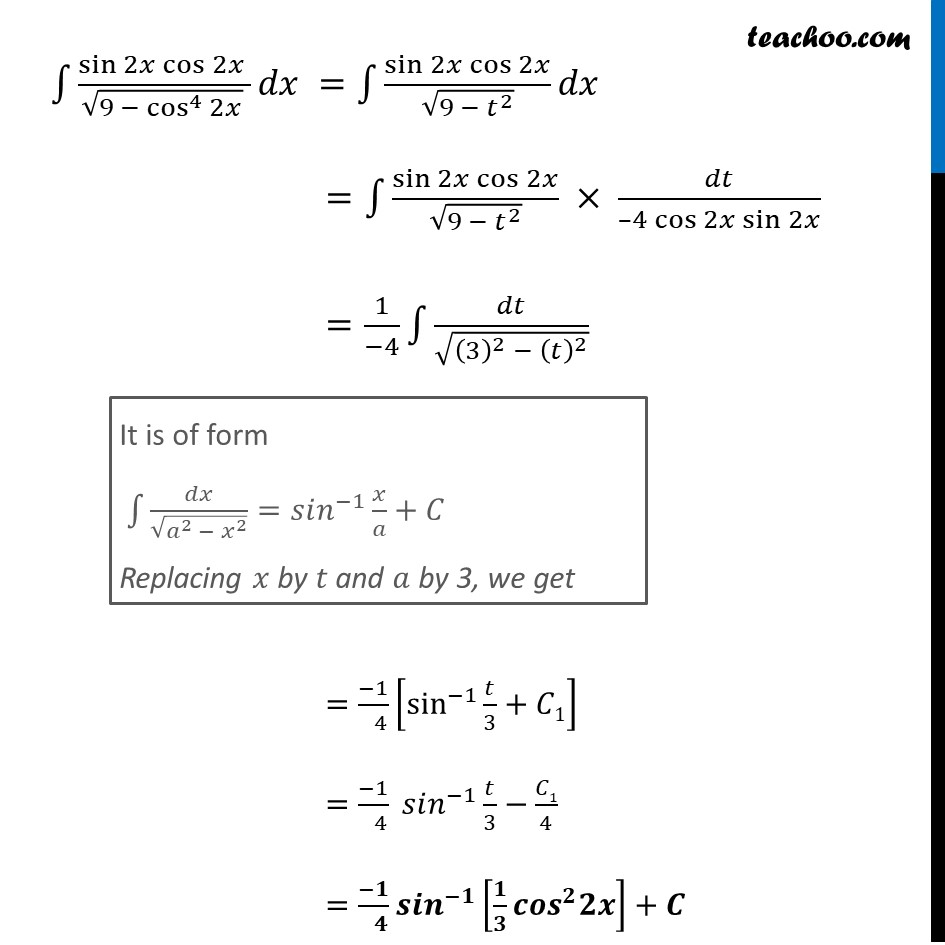Integration by specific formulaes - Formula 5

Chapter 7 Class 12 Integrals
Concept wiseLearn in your speed, with individual attention - Teachoo Maths 1-on-1 Class

### Transcript

Example 40 Evaluate ∫1▒(sin⁡〖2𝑥 cos⁡2𝑥 〗 𝑑𝑥)/(√(9 −cos^4⁡(2𝑥) ) ) 𝑑𝑥 ∫1▒(sin⁡〖2𝑥 cos⁡2𝑥 〗 𝑑𝑥)/(√(9 − cos^4⁡2𝑥 ) ) 𝑑𝑥 Let cos^2⁡2𝑥=𝑡 Differentiating both sides w.r.t.𝑥 –2.2 cos⁡2𝑥 sin⁡2𝑥=𝑑𝑡/𝑑𝑥 𝑑𝑥=𝑑𝑡/(–4 cos⁡2𝑥 sin⁡2𝑥 ) Hence, our equation becomes ∫1▒(sin⁡〖2𝑥 cos⁡2𝑥 〗 )/(√(9 − cos^4⁡2𝑥 ) ) 𝑑𝑥 =∫1▒〖sin⁡〖2𝑥 cos⁡2𝑥 〗/√(9 − 𝑡^2 ) 𝑑𝑥〗 =∫1▒〖(sin⁡2𝑥 cos⁡2𝑥)/√(9 − 𝑡^2 ) × 𝑑𝑡/(–4 cos⁡2𝑥 sin⁡2𝑥 )〗 =1/(−4) ∫1▒𝑑𝑡/√((3)^2 − (𝑡)^2 ) =(−1)/( 4) [sin^(−1)⁡〖𝑡/3+𝐶1〗 ] =(−1)/( 4) 𝑠𝑖𝑛^(−1) 𝑡/3−𝐶1/4 =(−𝟏)/( 𝟒) 𝒔𝒊𝒏^(−𝟏) [𝟏/𝟑 𝒄𝒐𝒔^𝟐 𝟐𝒙]+𝑪